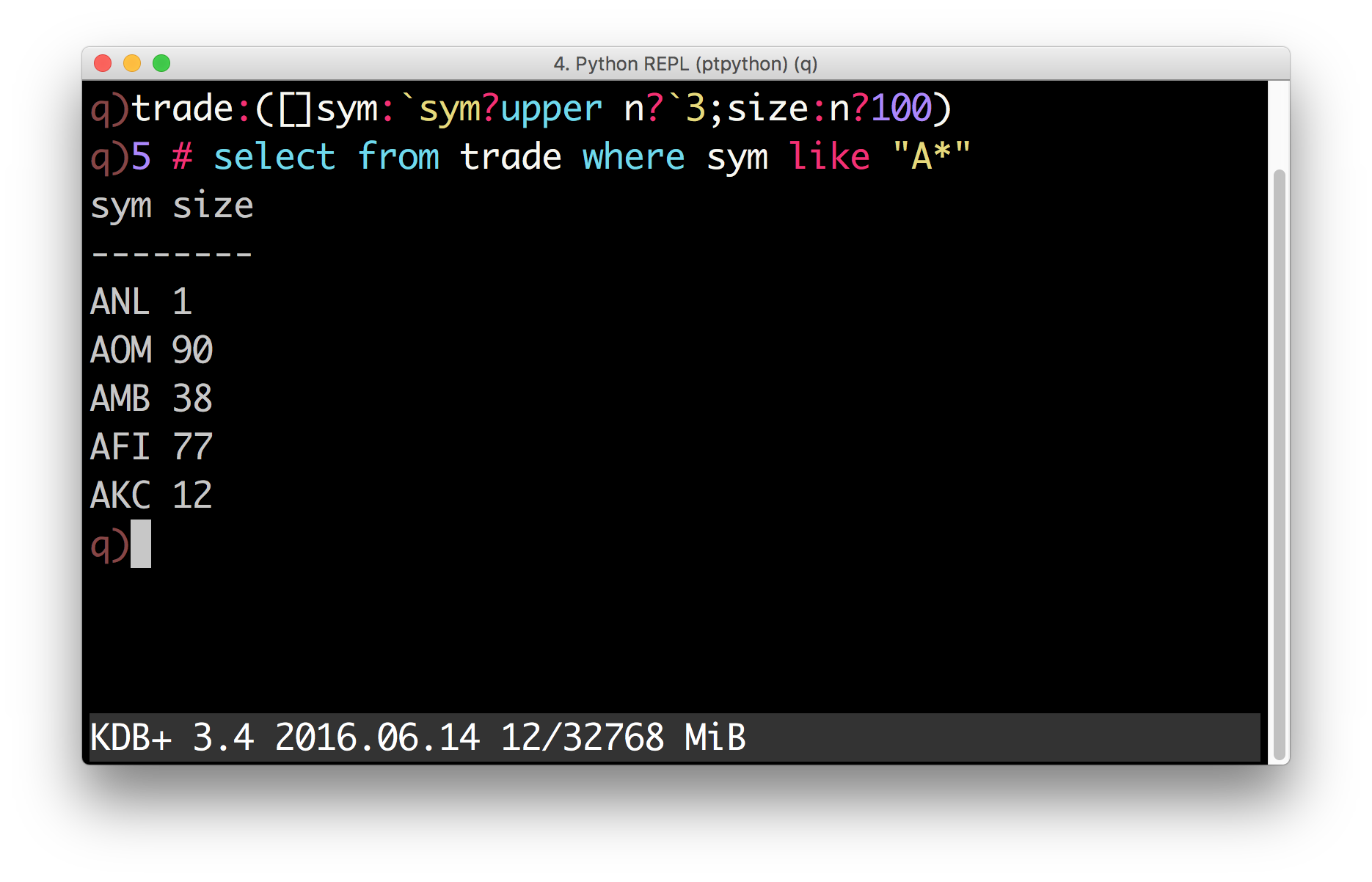# What’s New in PyQ 4.0¶

Release: 4.0 March 02, 2017

## Summary – Release highlights¶

• Enhanced q) prompt with syntax highlighting.
• New operators: <<, >> and @.
• Improved means for constructing K objects of arbitrary types.
• Type casts using attribute syntax.
• Improved numpy interoperability.
• Restored support for KDB+ 2.x.
• Better documentation.
• More k.h functions are exposed to Python internally.
• Added convenience scripts for starting different interactive sessions.
• Additional conversions between K and native Python objects.

## Enhanced q) prompt¶

The q) prompt will now use the prompt toolkit when available to provide a separate command history, q syntax highlighting and a status bar displaying system information.## New operators¶

Three new operators are defined for K objects: <<, >> and @.

### Shift operators¶

Shift operators << and >> can now be used to shift elements in K lists:

>>> q.til(10) << 3
k('3 4 5 6 7 8 9 0N 0N 0N')
>>> q.til(10) >> 3
k('0N 0N 0N 0 1 2 3 4 5 6')


### The @ operator¶

Users of Python 3.5 or later can now use the new binary operator @ to call q functions without using parentheses:

>>> q.til @ 5
k('0 1 2 3 4')


The same operator between two functions creates a function composition. For example, the dot product can be defined succinctly as

>>> dot = q.sum @ q('*')
>>> dot([1, 2, 3], [3, 2, 1])
k('10')


## Typed constructors and casts¶

Atoms and lists of like atoms can now be constructed from Python objects using typed constructors. For example, by default, a list of strings passed to the default K constructor becomes a symbol list:

>>> colors = K(['white', 'blue', 'red'])
>>> colors
k('whitebluered')


If you want to create a list of strings, you can use a typed constructor:

>>> K.string(["Donald E. Knuth", "Edsger W. Dijkstra"])
k('("Donald E. Knuth";"Edsger W. Dijkstra")')


If you already have a symbol list and want to convert it to strings, you can use the attribute access notation to perform the cast:

>>> colors.string
k('("white";"blue";"red")')


Similar operations can be performed with numeric data. For example, to create a matrix of single-precision floats (real), call

>>> m = K.real([[1, 0, 0],
...             [0, 1, 0],
...             [0, 0, 1]])
>>> m
k('(1 0 0e;0 1 0e;0 0 1e)')


To cast the result to booleans — access the boolean attribute:

>>> m.boolean.show()
100b
010b
001b


Unlike q, Python does not have special syntax for missing values and infinities. Those values can now be created in PyQ by accessing na and inf attributes on the typed constructors:

>>> for x in [K.int, K.float, K.date, K.timespan]:
...     print(x.na, x.inf)
0Ni 0Wi
0n 0w
0Nd 0Wd
0Nn 0Wn


## Interoperability with NumPy¶

### Matrices and arrays of higher dimensions¶

Arrays with ndim > 1 can now be passed to q and they become nested lists. For example:

>>> q.x = numpy.arange(12, dtype=float).reshape((2, 3, 2))
>>> q.x
k('((0 1f;2 3f;4 5f);(6 7f;8 9f;10 11f))')


Similarly, ndim > 1 arrays can be constructed from lists of regular shape:

>>> numpy.array(q.x)
array([[[  0.,   1.],
[  2.,   3.],
[  4.,   5.]],

[[  6.,   7.],
[  8.,   9.],
[ 10.,  11.]]])


### Times, dates and timedeltas¶

Prior to 4.0, conversion of temporal data to NumPy arrays would expose internal integer values. For example, a list of months

>>> months = q('2001.01m + til 3')


would become an integer array when converted to NumPy:

>>> numpy.array(months).tolist()
[12, 13, 14]


Now, an array of type datetime64 is returned:

>>> numpy.array(months)
array(['2001-01', '2001-02', '2001-03'], dtype='datetime64[M]')


Note that the resulting array has different numeric values and cannot share the data with the K object. To share the data and/or to get an array as in older versions, one should use the new data attribute:

>>> a = numpy.asarray(months.data)
>>> a.tolist()
[12, 13, 14]


An array constructed from the data attribute will use the same underlying storage. This means that changing the array will change the K object.

>>> a[:] += 998*12
>>> months
k('2999.01 2999.02 2999.03m')


### Complex numbers¶

Complex numbers can now be passed to and obtained from kdb+. When passed to kdb+, complex numbers are automatically converted to dictionaries with keys “re” and “im” and lists of complex numbers are converted to tables with columns “re” and “im”.

>>> q.z = [1 + 2j, 3 + 4j, 5 + 6j]
>>> q.z.show()
re im
-----
1  2
3  4
5  6
>>> [complex(x) for x in q.z]
[(1+2j), (3+4j), (5+6j)]


### Path objects¶

Path objects can now be used where q path handle symbols are expected

>>> import pathlib
>>> path = pathlib.Path('xyz')
>>> q.set(path, 42)
k(':xyz')
>>> q.get(path)
k('42')


### Named tuples¶

Named tuples are now converted to dictionaries:

>>> from collections import namedtuple
>>> Point = namedtuple('Point', 'x,y')
>>> q.point = Point(1, 2)
>>> q.point
k('xy!1 2')


As a consequence, a uniform list of named tuples is converted to a table:

>>> q.points = [Point(1, 2), Point(3, 4), Point(5, 6)]
>>> q.points.show()
x y
---
1 2
3 4
5 6


Adverbs can now be used on functions with different ranks. For example, scan and over can be used with monadic functions. To illustrate, the following code generates a Pascal triangle:

>>> f = q('{(0,x)+x,0}')
>>> f.scan(6, 1).show()
1
1 1
1 2 1
1 3 3 1
1 4 6 4 1
1 5 10 10 5 1
1 6 15 20 15 6 1


If only the last row is of interest – use over:

>>> f.over(6, 1)
k('1 6 15 20 15 6 1')